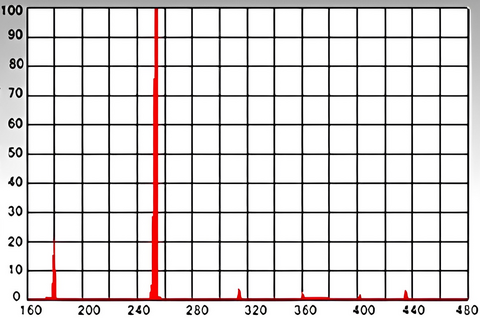UV Cleaning & Modifying

# About the wavelength of UV lamp light

About the wavelength of UV lamp light
In the case of ultraviolet cleaning and surface modification irradiation equipment, low-pressure mercury lamps that efficiently emit light at short wavelengths of 184.9 nm and 253.7 nm are generally used.
The shorter the wavelength of each wavelength of ultraviolet light, the higher the energy, with wavelengths of 184.9 nm and 253.7 nm capable of breaking the bonds of many organic materials.

## Spectral energy distribution of low-pressure mercury lamps## Relationship between wavelength and energy of light

The relationship between lamp wavelength and light energy is expressed by the following equation.

$E=\frac{\mathrm{hc}}{\lambda }$

 E ： Energy J h ： Planck’s constant　6.6260710-34 Js c ： Speed of light in vacuum　299792458 m/sec λ ： Wavelength m

※Planck's constant: A constant of proportionality derived from Max Planck, the founder of quantum mechanics, that expresses the proportional relationship between the energy possessed by a photon and its frequency of oscillation.

From this equation, the energy that low-pressure mercury lamps has at the main wavelengths of 184.9 nm and 253.7 nm, respectively, can be obtained as follows.

647 kJ/mol (6.7 eV) at a wavelength of 184.9 nm
472 kJ/mol (4.9 eV) at a wavelength of 253.7 nm

The binding energies of typical molecules are shown in "Table 1: Typical Molecular Binding Energies." The light energy from the low-pressure mercury lamps can cut molecular chains, and together with the effect of ozone generated at the same time, cleaning and surface modification effects can be expected.

Table1 Binding energies of typical molecules
Binding molecule Binding energies Binding molecule Binding energies
O-O 138.9 kJ/mol C-H 413.4 kJ/mol
C-N 291.6 kJ/mol H-Cl 431.8 kJ/mol
C-Cl 328.4 kJ/mol C-F 441.0 kJ/mol
C-C 347.7 kJ/mol O-F 462.8 kJ/mol
C-O 351.5 kJ/mol O=O 490.4 kJ/mol
Si-O 369.0 kJ/mol H-F 563.2 kJ/mol
N-H 390.8 kJ/mol C=C 607.0 kJ/mol

As shown in the formula for the relationship between wavelength and light energy, the shorter the wavelength, the greater the amount of energy, and the more effective the surface modification, but the restrictive conditions such as the distance light can reach in the general atmosphere and the price of the equipment increase.

For example, the excimer cleaning surface modification system (main wavelength: 172 nm) can modify the surface of polymeric materials with high molecular binding energy.

close